# Parallelism in Practice

## Approaches to Parallelism in Bioassays

This is our pre-publication version of our paper that examines the common methods used for evaluation of parallelism, and provides a guide to choosing the optimal method taking into account factors such as data available, regulatory environment and expected data variability. A flow chart guide is included.

The paper was later published in the Journal of Pharmaceutical Science and Technology

# Paper Abstract

Relative potency bioassays are used to estimate the potency of a test biological product relative to a standard or reference product. It is established practice to assess the parallelism of the dose response curves of the products prior to calculating relative potency. This paper provides a review of parallelism testing for bioassays. In particular three common methods for parallelism testing are reviewed: two significance tests (the F-test, the $$\chi^2$$ -test) and an equivalence test. Simulation is used to compare these methods. We compare the sensitivity and specificity and ROC curves, and find that both the $$\chi^2$$ -test and the equivalence test outperform the F-test on average, unless the assay-to-assay variation is considerable. No single method is optimal in all situations. We describe how bioassay scientists and statisticians can work together to determine the best approach for each bioassay, taking into account its properties and the context in which it is applied.

Fleetwood K, Bursa F, Yellowlees A. Parallelism in Practice: Approaches to Parallelism in Bioassays. PDA Journal of Pharmaceutical Science and Technology. 2015 Mar 1;69(2):248-63.

# 1 Introduction

The relative potency (RP) of a test product is defined as the ratio of the dose of a standard product to the dose of the test product such that both products have the same biological effect.   An important concept in bioassays is similarity. Two products are said to be similar if they act as dilutions of the same substance; this is equivalent to the two products having parallel dose response curves (Finney 1964).  If this is the case then the RP can then be estimated from the (constant) horizontal distance between the dose response curves.

Parallel and non-parallel dose response curves are illustrated in Figure 1. Part (a) illustrates parallel curves: regardless of the biological effect (in this case intensity) the distance between the curves is always the same. Hence the estimate of RP is constant. Part (b) illustrates non-parallel curves: as the intensity increases the difference between the curves widens: the estimate of RP varies with dose.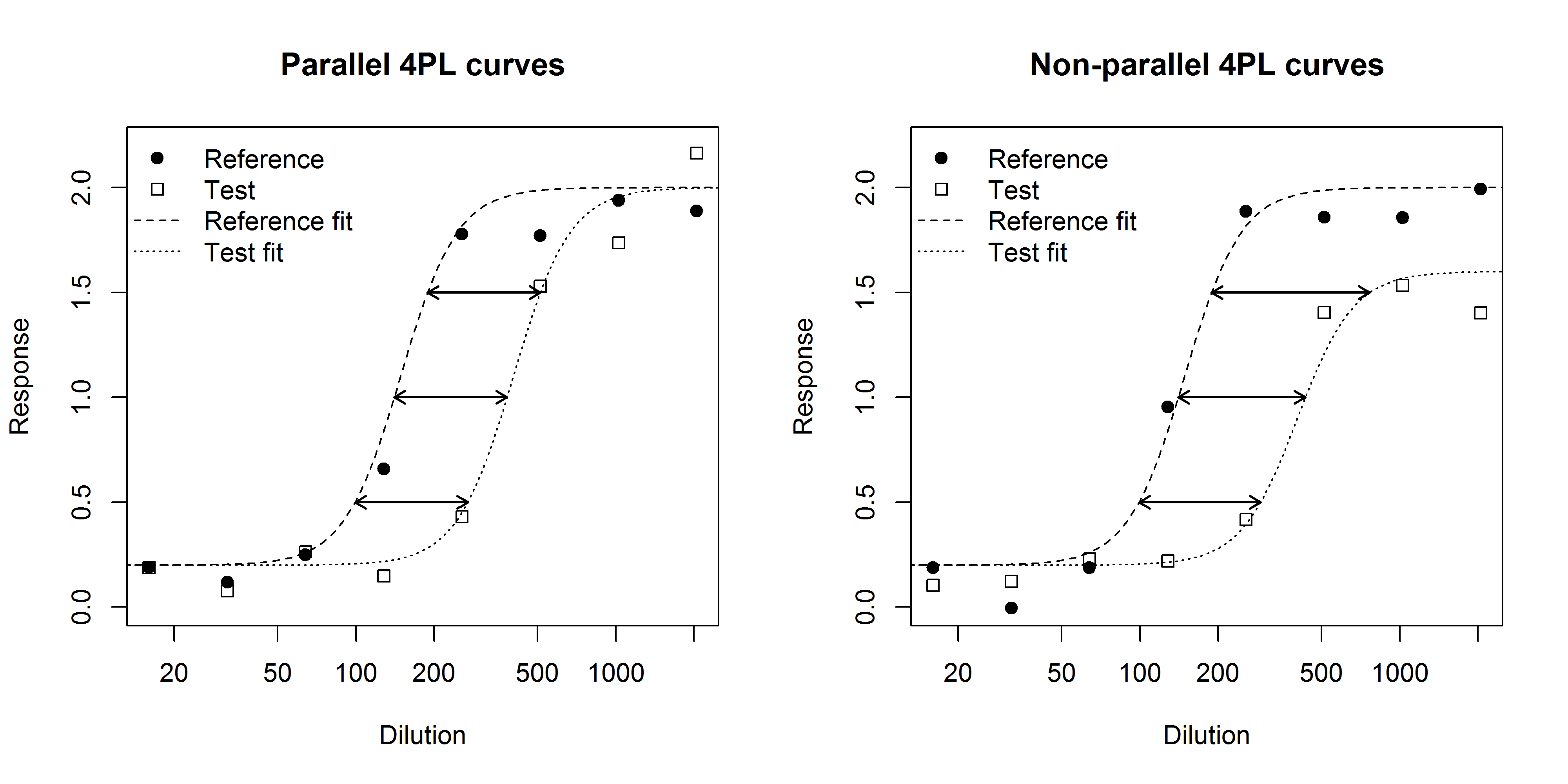Figure 1: An illustration of parallel (a) and non-parallel (b) 4PL dose-response curves.

There has recently been much debate about the best way to assess parallelism in bioassay.

There are two distinct philosophical approaches.  In the first, similarity is assumed and evidence to contradict this assumption is sought.  Thus evidence of lack of parallelism can be taken to prove dissimilarity but lack of sufficient evidence cannot be taken to prove similarity.  Tests following this approach are sometimes referred to as ‘difference tests’.  The F-test preferred by the European Pharmacopoeia guidelines (Council of Europe 2011, Finney 1964) is one such test.  It has been criticized as over-sensitive to small deviations from parallelism in highly precise assays (Callahan and Sajjadi 2003, Hauck et al. 2005, Gottschalk and Dunn 2005a). Gottschalk and Dunn (2005a) proposed an alternative approach that uses weighting of the responses followed by a  test.

The second approach assumes lack of similarity, and seeks evidence to prove similarity.  This approach has been advocated by Callahan and Sajjadi (2003) and Hauck et al. (2005).  It is recommended by the United States Pharmacopeia (USP) bioassay guidelines (The United States Pharmacopeial Convention 2012a, 2012b, 2012c).  Tests following this approach are sometimes referred to as ‘similarity tests’ or ‘equivalence tests’.

In this paper these approaches to parallelism testing are reviewed and compared with respect to their performance. Simulations are used to evaluate which approach works best in different situations. The intended audience for this paper is bioassay scientists. Statistical detail is kept to a minimum; references are provided for texts that provide more technical information.

# 2 Models for bioassay data

A parallel-line bioassay measures a response for a series of concentrations of the standard and test products. This response may be a continuous measurement such as luminosity or survival time, or a binomial outcome, for example, dead or alive. Bioassay statistical analysis involves fitting a model to the dose-response relationship. The type of model depends on the characteristics of the assay.  Standard linear models or more complex models such as four and five parameter logistic models are common for continuous data (Gottschalk and Dunn 2005b) and generalized linear models for binary responses (McCullagh and Nelder 1989) are important.

In this paper we focus on standard linear models.  These fit a straight line to the concentration and response data for each product. The straight lines are described by their intercepts and slopes. A standard and a test product are similar if they have the same slope.  We also refer to four parameter logistic models, described by lower and upper asymptotes, a slope and a mid-point or EC50; here a standard and test product are similar if they have the same slope, lower asymptote and upper asymptote.

# 3 Three tests for parallelism

We will consider, in the context of the standard linear model, the two ‘difference tests’ mentioned above (the F-test and the chi-squared test) and an ‘equivalence test’ based on the difference between the slopes of the two dose response lines. We will also discuss three variants that have been suggested, a weighted F test, a weighted equivalence test, and an equivalence test with fixed ‘equivalence interval’.  Specificity and sensitivity of the tests are considered.

(a) F-test:  This is a classical statistical test for comparing two models.  The underlying assumption is that the variance is constant across all groups within the assay. The F-statistic is defined as:

$$\left ( \frac{\text{RSS}_p – \text{RSS}_q}{q-p}\right ) / \left ( \frac{\text{RSS}_q}{n-q} \right ) \sim F_{q-p; n-q}$$

where RSS? and RSSq are the residual sums of squares of the parallel and non-parallel models (with p and q parameters)  respectively, and n is the number of observations. The statistic has the $$F_{q-p; n-q}$$  distribution only if the variance of the response is constant across the range of the assay, for both test and reference material, and if the response is normally distributed.  The analyst needs to set the significance level (usually 5%).  Note that the probability of correctly concluding parallelism, is given by (100% – test level, usually 95%).

(b) Chi-squared test: Here the response variable must be weighted by the inverse of its variance. The test statistic is:

$$\text{RSS}_p – \text{RSS}_q$$

which has a $$\chi^2_{q-p}$$ distribution only if the model has been correctly weighted such that the variance of the weighted response is 1 across the range of the assay, for both test and reference material, and if the response is normally distributed.  A set of historical assays must be used to estimate the relationship between the response and its variance; this provides the weighting. The analyst also needs to set the significance level (usually 5%).  Again, the probability of correctly concluding that truly non-parallel curves are non-parallel depends on the true difference to be detected, and on the underlying variance of the data.

(c) An equivalence test:  For a linear model the null hypothesis is that some measure of the difference, ∆, between the standard and reference slopes is greater than a pre-specified value; the alternative hypothesis is that the difference is less than the pre-specified value.  A model with separate slopes for the standard and test products is fitted to the data. This provides an estimate of ∆ and a confidence interval (CI) for ∆.  If the CI lies within a pre-specified ‘equivalence interval’ then parallelism is demonstrated. The analyst needs to define the ‘equivalence interval’ by setting the confidence level for the interval (usually 95%), which is equivalent to the probability of correctly concluding parallelism when the true difference between the slopes is zero.

For the purposes of this study we have chosen the difference between slopes as the measure of difference, ∆.  We set the equivalence interval based on historic data (a common approach) as the interval which includes 95% of intervals generated from data known to be truly parallel.

An alternative is to set the limits independently of the data; for example 80% to 125% for slope ratio, or using knowledge of what an acceptable level of non-parallelism is for the assay. For this study we investigate this approach by (arbitrarily) setting the equivalence interval to be a difference of slopes of less than 0.4.

For all the approaches except the equivalence test with fixed limits, the probability of correctly concluding that truly parallel lines are parallel is set, either by the level of the test or the confidence level for the interval. The probability of correctly concluding that non-parallel curves are non-parallel, for a given true difference in slopes, depends on the noise in the assay responses. For the equivalence test with fixed limits, however, both the probability of correctly concluding that truly parallel lines are parallel and the probability of correctly concluding that non-parallel curves are non-parallel depend on the noise in the assay responses.

# 4 Comparison of tests under different variance patterns

One way to understand how the three approaches differ is to consider how they estimate the variance used (implicitly or explicitly) to decide whether the observed value of the test statistic is significant or not.

• For the F-test, the variance is estimated from the within-assay variance of the current assay only. It takes no account of historic data.
• For the $$\chi^2$$ test, the variance is estimated from historical within-assay data only.  The test statistic is composed of a function of weighted observations which are assumed to have unit variance.
• For the equivalence test, both the historical within-assay and assay-to-assay variance contribute to the equivalence interval. In addition, the CI for the assay being tested will be a multiple of the current assay standard deviation.

The variance estimates used in each approach, including the weighted versions of the F-test and equivalence test, are summarised in Table 1.

Table 1: Sources of variance estimates in the approaches to parallelism testing

 Includes variance estimate from: Historical mean variance Historical within-assay Historical  between-assay Current-assay mean variance Within-current-assay F test No No No Yes No $$\chi^2$$ test Yes Yes No Yes Yes Equivalence Yes No Yes Yes No Weighted F test No Yes No Yes Yes Weighted equivalence Yes Yes Yes Yes Yes

Figure 2 illustrates three possible patterns of variation.  The variance can be either independent of dose (as in Scenarios A and B) or increasing with dose (Scenario C).  The variance may also vary from assay to assay (Scenario B).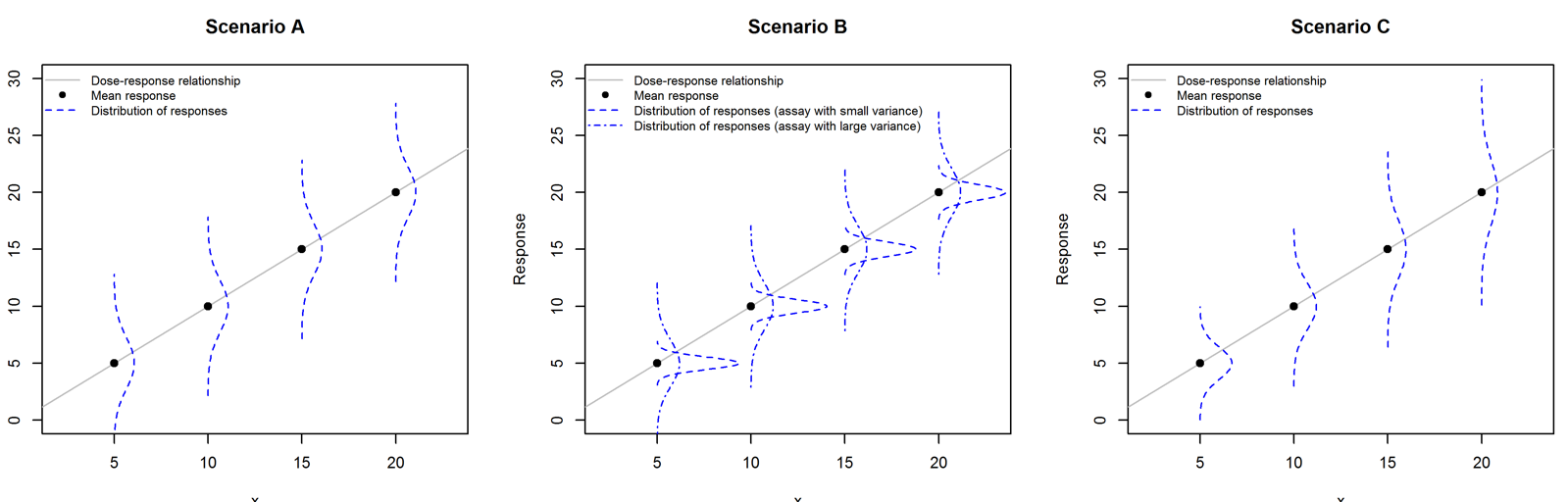Figure 2: Different variance behaviours, within- and between-assays, as used in the simulated scenarios. In Scenario A, there is constant within-assay variance, which is the same for all assays. In Scenario B, some assays have larger variance than others (two example assays are shown as dashed and dot-dashed lines). In Scenario C, the within-assay variance increases with the response, but is the same for all assays. Specifically, the error term is normally distributed,$$e_{ij} \sim N(0,\sigma^2_{ij})$$, with $$\sigma^2_{ij} = \mathcal{S}_{i}^2 + \mathcal{T}_{j}^2$$ .    $$\mathcal{S}_{i}^2$$ varies within-assay and depends on the response $$\mathcal{Y}$$, and $$\mathcal{T}^2_{j}$$ varies between assays; $$\mathcal{T}^2_{j} \sim lnN(\mu_T,\sigma_{T} )$$. The parameters $$\mathcal{S}^2_{i}, \mu_T,$$ and $$\sigma_{T}$$ are shown in tables 2 and 3 for each scenario.

# 5 Parallelism testing on simulated data sets

We have used simulation to investigate the three approaches in scenarios where we know whether the lines are truly parallel or not.  We base our simulation on a bioassay where 4 doses, equally spaced on the log scale, are used, with 10 independent observations per dose. We assume the response is normally distributed with mean which is related linearly to the log dose.  The variances have been constructed to match Scenarios A, B and C as shown in Figure 2.

We then conducted the following simulation study, based on a reference material for which the dose-response relationship was a straight line with slope of 1 unit, and the test material dose-response relationship was either parallel to the reference (slope of 1 unit) or non-parallel (slope of 1.25 units).  It is assumed that this slope difference represents an important lack of parallelism which should not be acceptable.   For each variance scenario:

1. To mimic a historical data set, we simulated a number of parallel assays. Using these we estimated:

(a) the weights needed for the chi-squared test, by fitting a response-variance relationship with a power-law fit (Gottschalk and Dunn 2005a) and

(b) the equivalence interval for the equivalence test, by extracting 95% CIs on the difference of slopes, taking the absolute values of the further point from zero, and then ranking these and setting the equivalence limit as the 95th percentile of these values.

1. We then simulated

(a) a further 1,000 parallel assays and

(b) a further 1,000 non-parallel assays.

For each of the 2,000 simulated assays we calculated the three test statistics described above.  In addition, we used weighted fits for the F-test and the equivalence test to explore how these perform, weighted by the inverse of the fitted response-variance relationship, as for the chi-squared test.

This process was repeated 10 times with the ‘historical’ data set containing 20 assays, and a further 10 times with the ‘historical’ data containing 100 assays.  Figure 3 shows examples of parallel and non- parallel simulations for variance scenario A.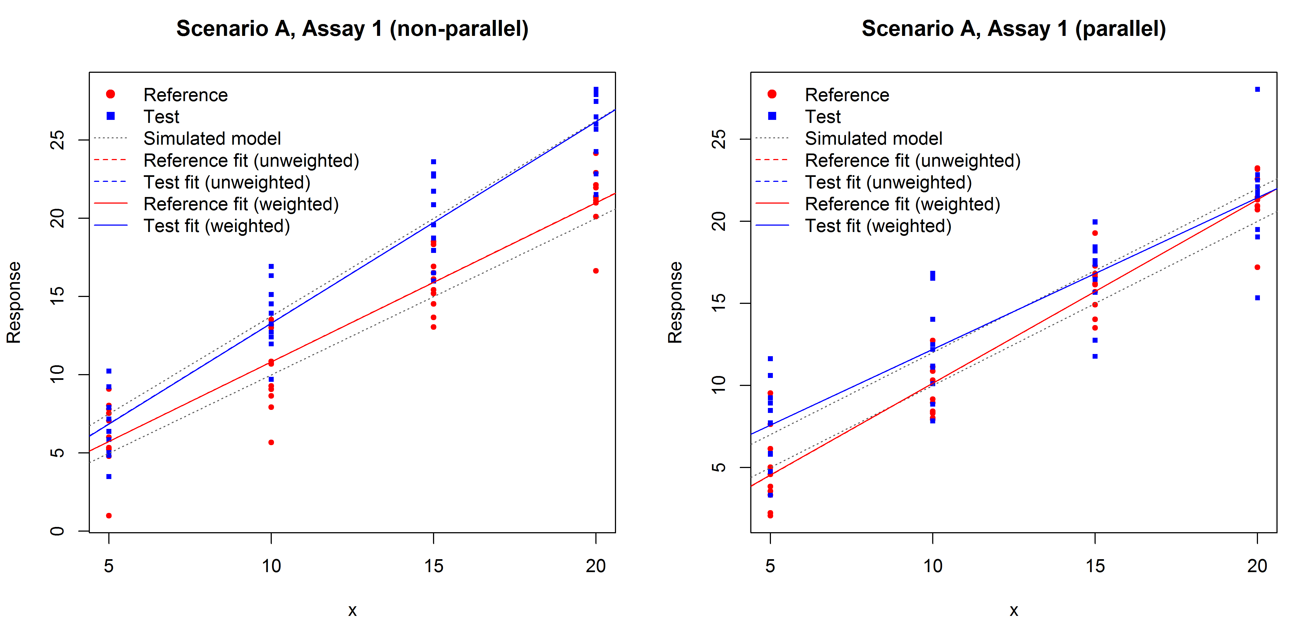Figure 3: Examples of parallel and non-parallel assays in the simulation.

We show the percentage of truly parallel assays correctly identified, and the percentage of truly non-parallel assays correctly identified, for each method for each scenario in Tables 2 and 3.  Note that the proportion of truly parallel assays that are classified as parallel should be on average 95% by definition in all cases except the equivalence test with fixed equivalence limits.

Figure 4, Figure 5 and Figure 6 illustrate the degree of agreement amongst the methods for the three scenarios, in the case where the historical data set consisted of 100 assays.  In these figures the solid lines relate to the $$\chi^2$$  test and the F-test, indicating p = 5%; assays lying below or to the left of this line are classified as non-parallel.  The dashed line relates to the equivalence test based on historical data: the maximum of the absolute values of the confidence limits is compared with this and assays lying above this value are classified as non-parallel. Finally, the dotted line shows the fixed equivalence limit: again, the maximum of the absolute values of the confidence limits is compared with this and assays lying above this value are classified as non-parallel.

Table 2: Summary of results for simulations using a 20-assay training set

 Scenario A B C Across 10 training sets (each of 20 assays): Median Median Median (min-max) (min-max) (min-max) F-test True positives 79.10% 85.30% 72.10% (78.1-80.7%) (84.2-88.3%) (69.9-74.8%) True negatives 95.20% 95.30% 95.20% (93.2-95.8%) (94.3-96.1%) (93.7-95.7%) $$\chi^2$$-test True positives 86.80% 90.20% 79.00% (77.9-83.3%) (12.6-97.1%) (76.7-83.6%) True negatives 95.10% 96.00% 94.50% (92.4-96.1%) (88.4-98.6%) (92.6-96.5%) Equivalence True positives 86.40% 12.90% 87.50% (69.1-97.5%) (0.1-83.8%) (69.3-95.1%) True negatives 91.50% 95.10% 89.00% (62.4-97.6%) (82.6-99.7%) (74.6-96.9%) F-test (weighted fits) True positives 79.10% 85.20% 77.90% (78.2-80.6%) (84.2-88.2%) (76.3-80.5%) True negatives 95.00% 95.40% 95.00% (93.2-95.7%) (94.2-96.2%) (93.4-96.3%) Equivalence (weighted fits) True positives 86.20% 13.00% 89.90% (70.5-97.6%) (0.1-83.6%) (79.6-97.8%) True negatives 91.50% 94.90% 92.10% (62.1-97.5%) (83.1-99.7%) (71.0-97.4%) Equivalence (fixed limits) True positives 61.90% 31.00% 66.50% (60.6%-63.3%) (28.8%-34.0%) (64.2%-68.1%) True negatives 98.60% 91.50% 98.50% (98.0%-99.0%) (89.9%-93.1%) (98.2%-98.9%) Parameters $$\mathcal{S}^2_{i}$$ 5 $$0.01 \mathcal{Y}$$ $$0.4 \mathcal{Y}$$ $$\mu_T$$ -10 0.5 -10 $$\sigma_T$$ 0 1.5 0

Table 3: Summary of results for simulations using training sets with 100 assays

 Scenario A B C Across 10 training sets (each of 100 assays): Median Median Median (min-max) (min-max) (min-max) F-test True positives 79.00% 85.90% 72.10% (77.6-80.1%) (84.5-86.6%) (70.9-73.5%) True negatives 95.40% 94.70% 95.30% (93.5-96.1%) (94.1-96.0%) (93.7-95.9%) $$\chi^2$$-test True positives 80.10% 89.30% 79.30% (78.7-81.2%) (79.8-90.9%) (78.6-81.3%) True negatives 95.40% 94.90% 95.10% (92.8-95.9%) (92.7-96.7%) (93.4-95.8%) Equivalence True positives 81.70% 14.10% 84.70% (74.0-87.3%) (5.6-25.2%) (76.9-89.0%) True negatives 93.70% 94.90% 94.30% (89.8-96.7%) (92.2-96.8%) (87.5-97.1%) F-test (weighted fits) True positives 79.00% 86.10% 78.40% (77.6-80.1%) (84.6-86.9%) (76.3-80.1%) True negatives 95.40% 94.50% 95.10% (93.4-96.0%) (93.9-96.2%) (93.5-95.7%) Equivalence (weighted fits) True positives 81.40% 14.10% 89.20% (73.9-87.6%) (5.6-27.0%) (80.4-92.7%) True negatives 93.80% 94.90% 92.70% (89.8-96.8%) (92.0-96.8%) (90.4-97.4%) Equivalence (fixed limits) True positives 62.20% 31.90% 67.10% (60.5%-63.7%) (28.2%-33.7%) (66.1%-68.2%) True negatives 98.90% 91.40% 98.60% (98.2%-99.2%) (89.6%-92.1%) (98.0%-99.1%) Parameters $$\mathcal{S}^2_{i}$$ 5 $$0.01 \mathcal{Y}$$ $$0.4 \mathcal{Y}$$ $$\mu_T$$ -10 0.5 -10 $$\sigma_T$$ 0 1.5 0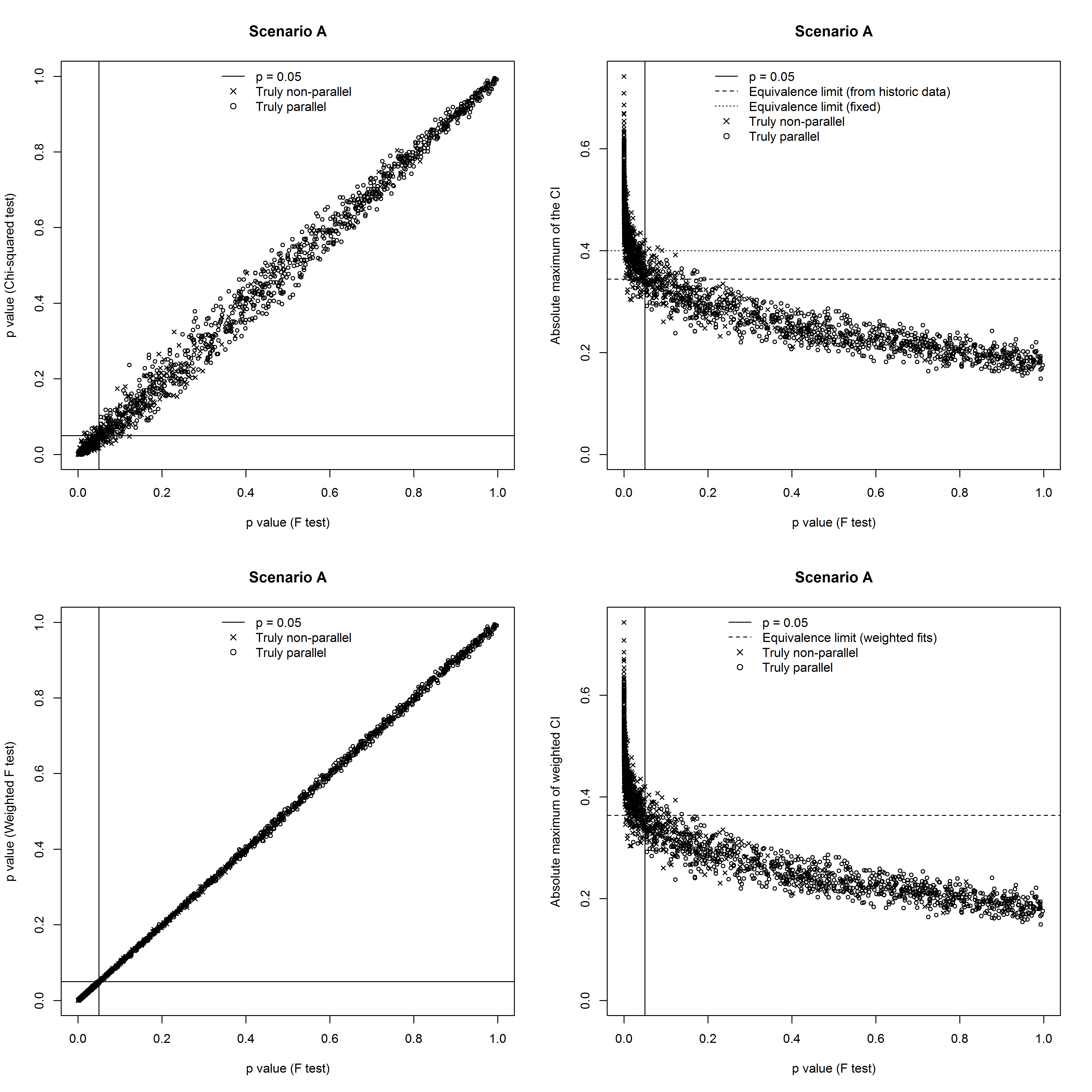Figure 4: Results in scenario A using a historical data set with 100 assays: Comparison of F-test and other approaches.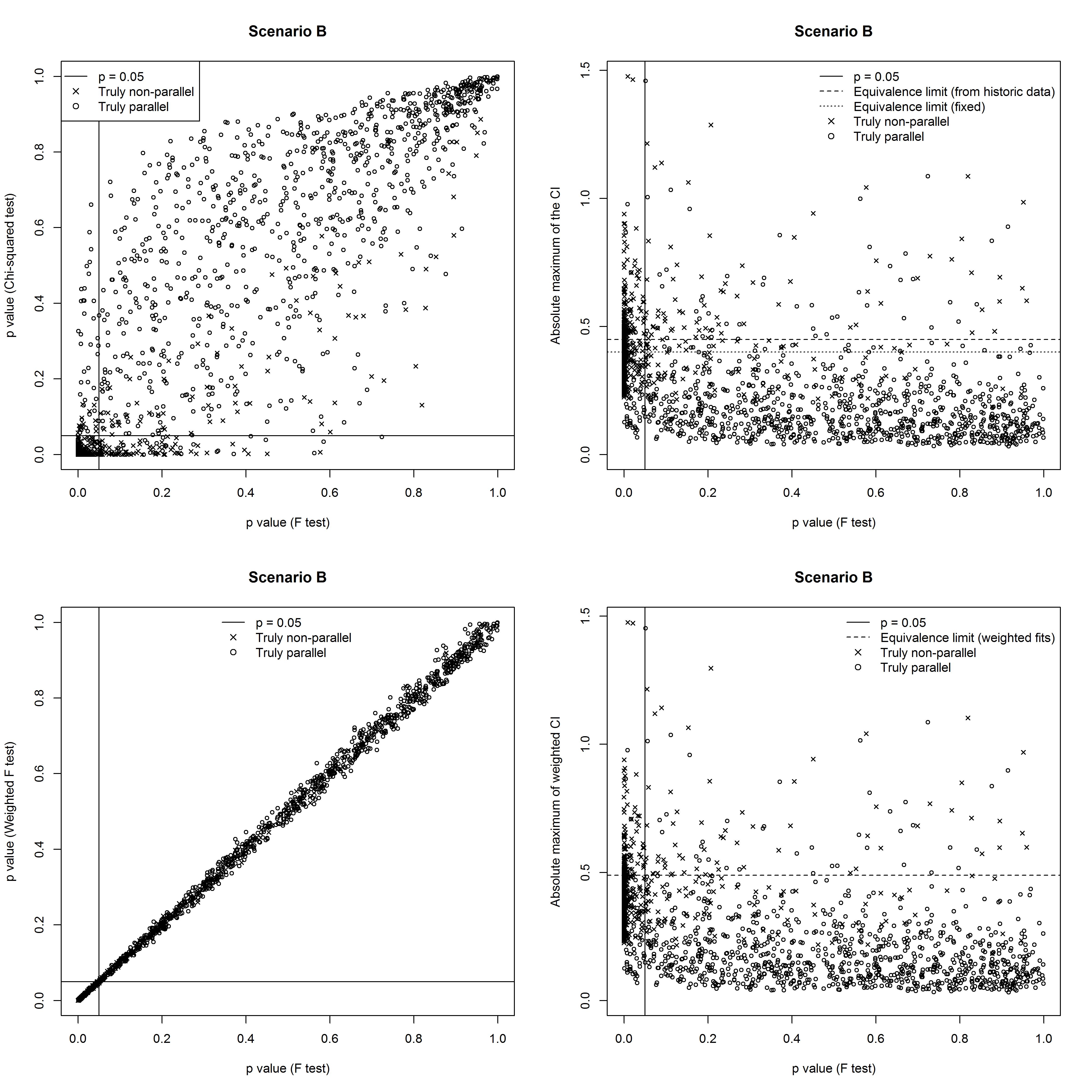Figure 5: Results in scenario B using a historical data set with 100 assays: Comparison of F-test and other approaches.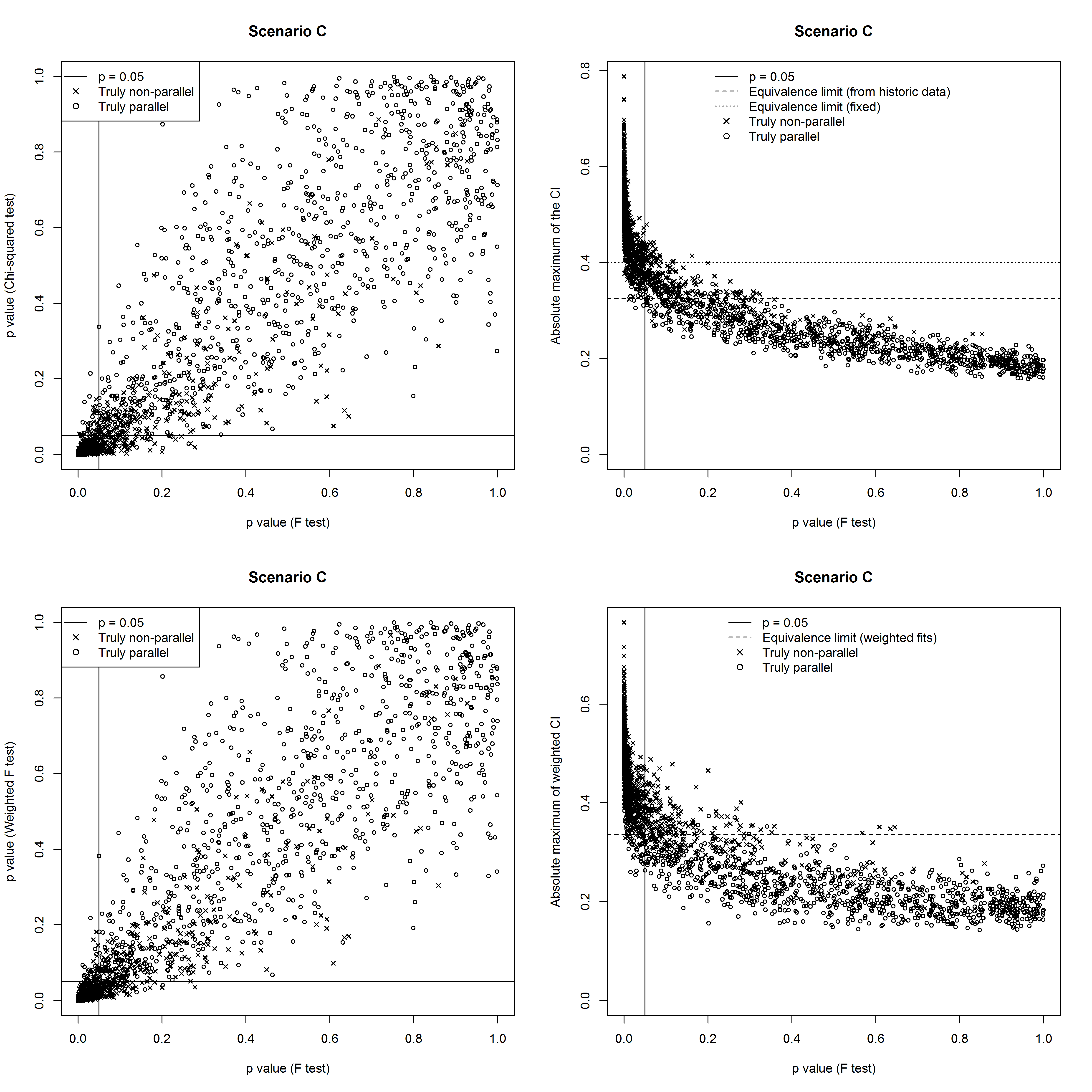Figure 6: Results in scenario C using a historical data set with 100 assays: Comparison of F-test and other approaches.

Figures 7, 8 and 9 show receiver operating characteristic (ROC) curves for the different methods for scenarios A, B and C respectively, for the case where there are 100 assays in the historical data set. In these figures sensitivity, the probability of correctly identifying non-parallel slopes, is plotted against 1-specificity, the probability of wrongly identifying parallel slopes as non-parallel. On each figure the curves for the 10 repeats are overlaid. On each curve the marked circle shows the sensitivity and specificity obtained with a significance level of 5% (for the F-test and $$\chi^2$$-test) or with the equivalence limit set to contain 95% of CIs in the historic data set (for the equivalence test). The square shows the sensitivity and specificity obtained with a fixed equivalence limit of 0.4. Moving left along the curves from these points corresponds to decreasing the significance level or increasing the equivalence limit; moving right corresponds to increasing the significance level or decreasing the equivalence limit.

Figure 7: ROC curves in scenario A using a historical data set with 100 assays. Filled blue circles, hollow blue circles, and filled green circles show sensitivity and specificity obtained with a significance level of 5% for the F-test, weighted F-test and $$\chi^2$$-test respectively. Filled and hollow orange circles show sensitivity and specificity obtained with the equivalence limit set to contain 95% of CIs in the historic data set for the equivalence test and weighted equivalence test respectively. Filled orange squares show sensitivity and specificity obtained with a fixed equivalence limit of 0.4.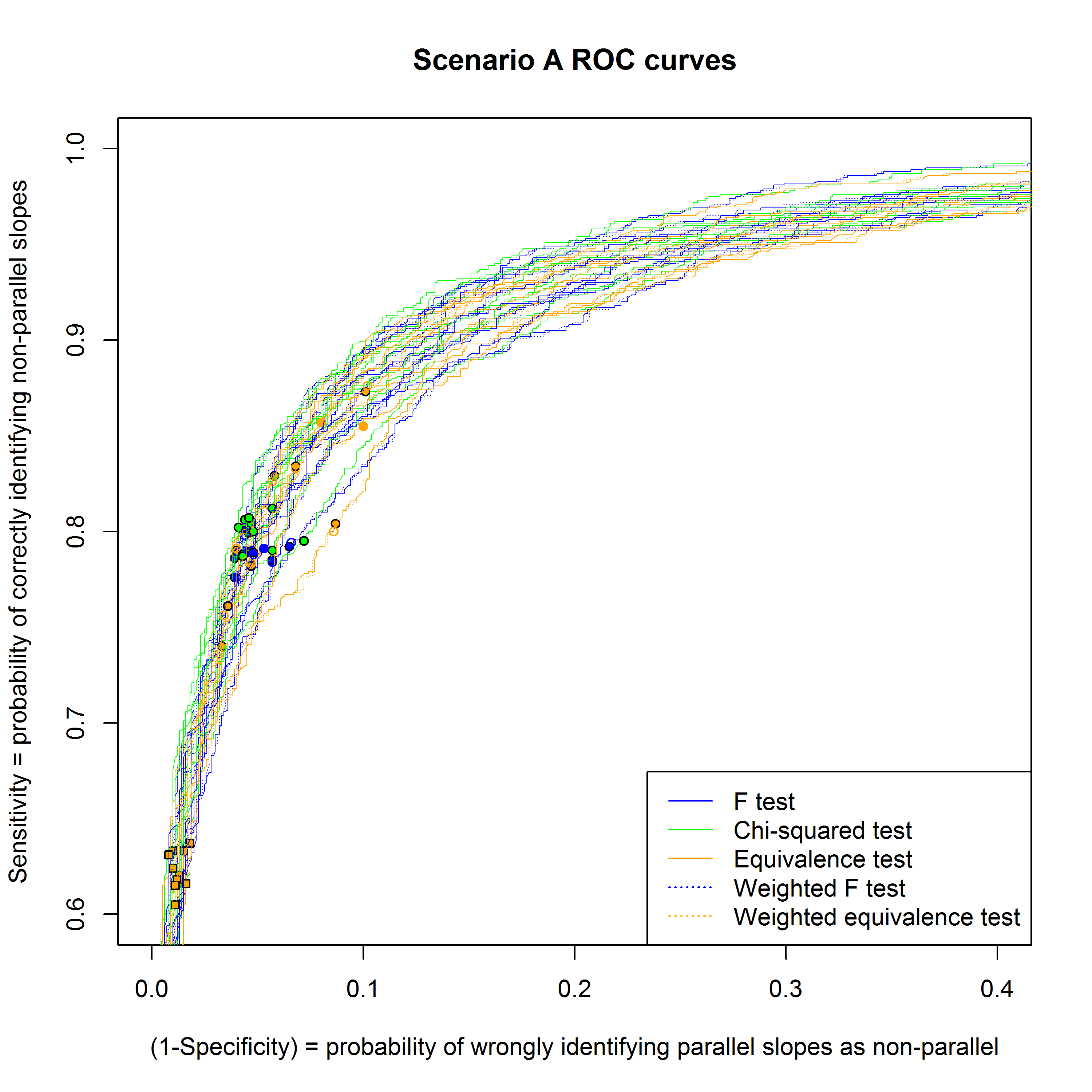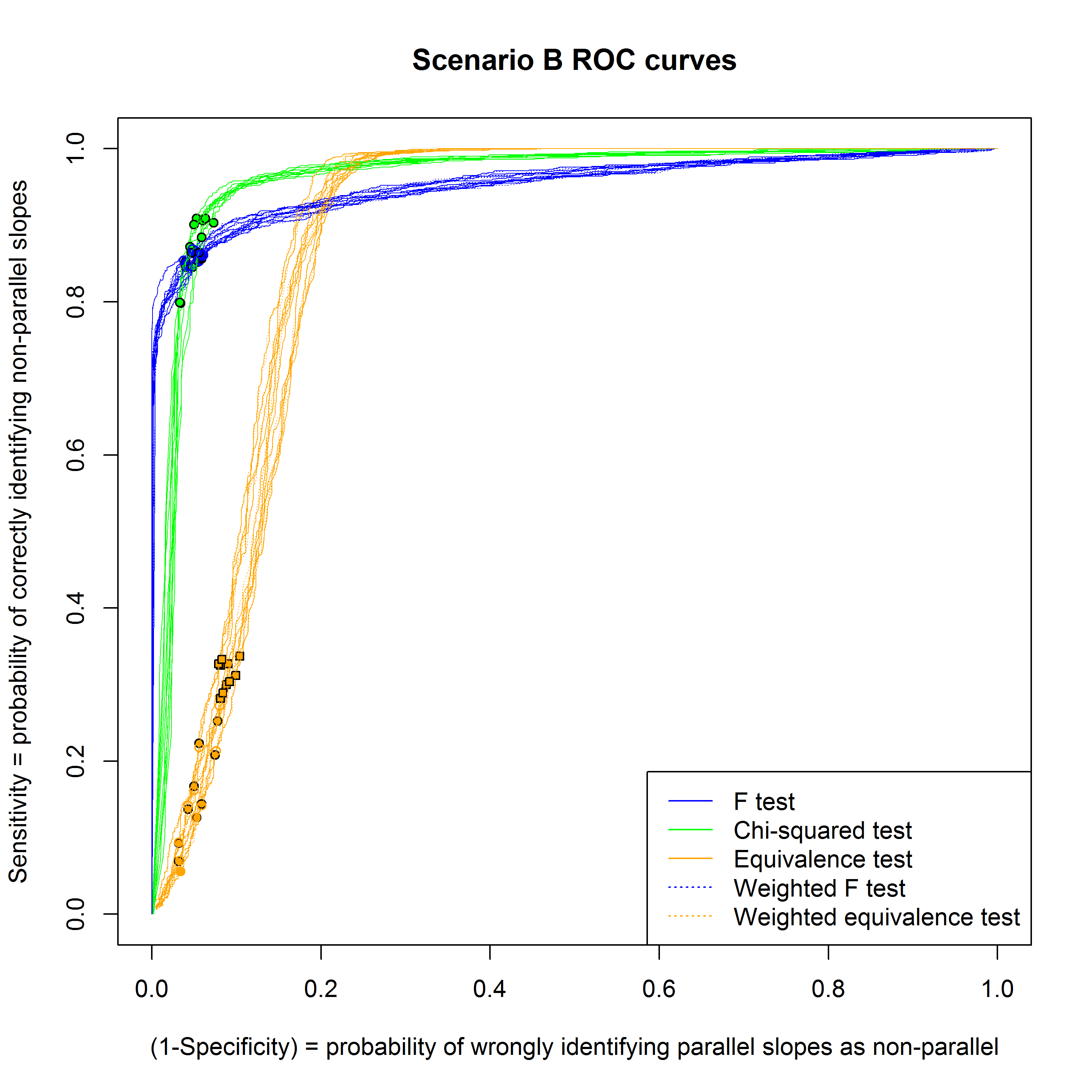Figure 8: As for Figure 7, for scenario B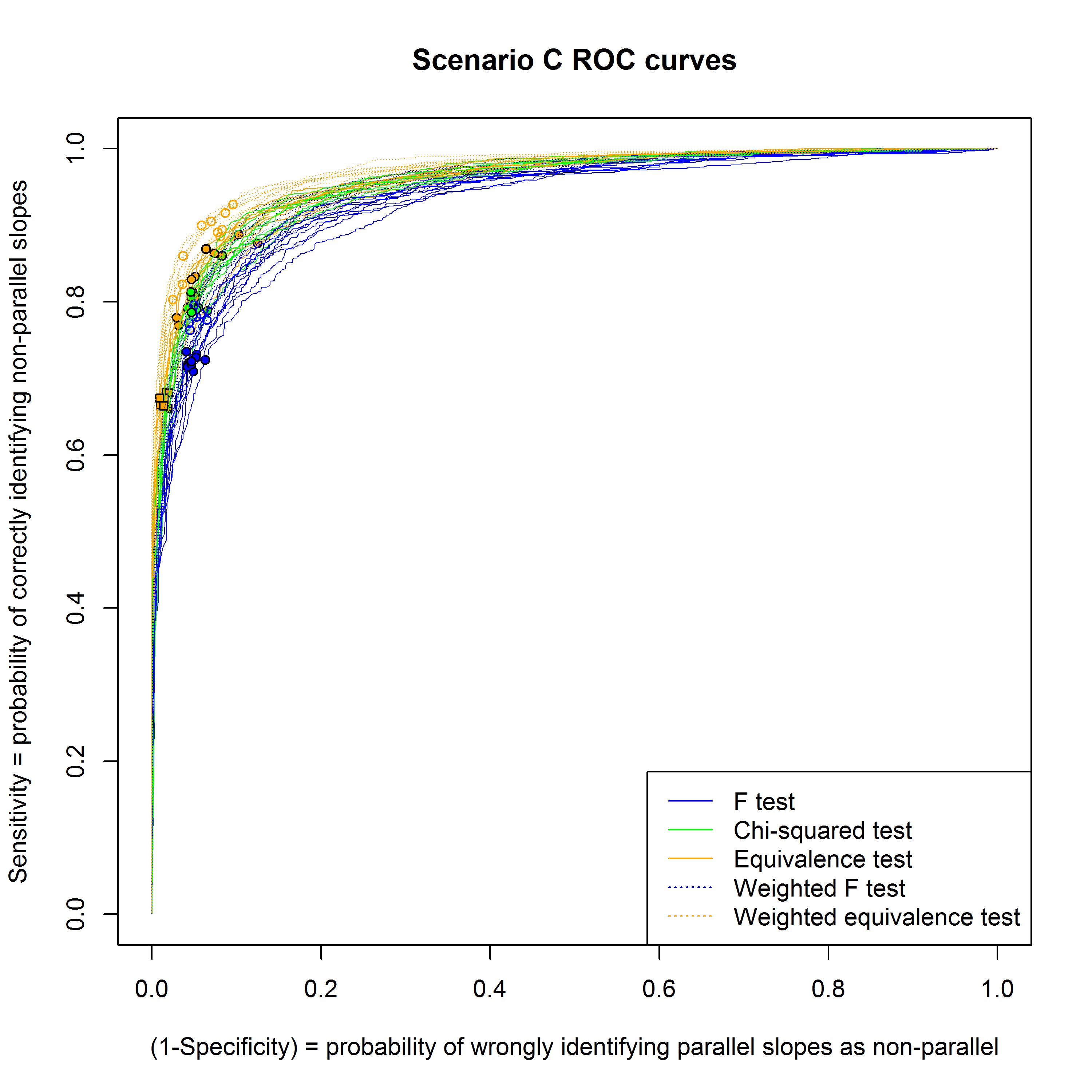Figure 9: As for Figure 7, for scenario C.

# 6 Comparing the approaches

The probability of a truly parallel assay being classified as such is consistent across all approaches, though the equivalence test is more variable in this regard, particularly with the smaller ‘historical’ data set.  The probability of a truly non-parallel assay being classified as such is much more variable.

Scenario A: Constant variance both within and between assays

All the approaches perform well, because the variance is constant.  A partial exception is the equivalence test based on historical data, where the number of correctly identified assays varies over a wider range. This is because it is very sensitive to the small number of assays with the largest absolute maxima of the CIs in the historical set, and an ‘unlucky’ historical set can easily cause the equivalence limit to be set too high or too low.  Figure 4 shows that the results of the different approaches are strongly correlated.

Scenario B: Variance constant within assay, varies strongly between assays

In this scenario the F-test works well, since the variance does not vary strongly across the doses within each assay and the F test does not consider between assay variance. The $$\chi^2$$-test (with small historical data set) and equivalence test are disadvantaged because the assay to assay component of the variance is large so their historical estimates of the variance is poor, impacting on the estimate for the weights for the former and the equivalence interval for the latter. The variability of the proportion of correctly classified non-parallel assays is very high when there are only 20 assays in the historical data set. There is much less variability when fixed equivalence limits are used since these do not rely on historical data.

Scenario C: Variance varies within each assay

Here the variance is linear in the response and constant between assays. The $$\chi^2$$-test works well, since it correctly uses weighted fits and its estimate of the variance from historical data is accurate. The other two approaches work less well, since they wrongly assume the variance is equal across doses. The weighted F-test and equivalence test perform better than their un-weighted counterparts – indeed, the weighted F-test is nearly as good as the $$\chi^2$$-test.

The approaches can also be compared by examining the ROC curves in Figures 7-9. Here the appropriateness of an approach can be assessed by how high the sensitivity is for a given specificity. For scenario A all approaches perform similarly, but this is not the case for scenarios B and C. In particular for scenario B which approach is best depends on the desired specificity: for high specificity the F test is best, for medium specificity the $$\chi^2$$-test is best, and for low specificity the equivalence test is best. The large scatter in the position of the points for the equivalence test using historical data is due to the variability of the equivalence limit, which can be easily affected by an ‘unlucky’ historical data set; this scatter is even larger for the ROC curves using 20 assays in the historical data set (not shown). Thus even when the equivalence test apparently performs well, as in scenario C, the variability means that the performance obtained in practice may be worse than for the other approaches, if an ‘unlucky’ historical data set is encountered. Using fixed equivalence limits avoids this problem; however the false negative rate may become larger than 5%, as in scenario B.

# 7 Discussion

The F-test and equivalence test have previously been compared by Jonkman and Sidik (2009). They considered the case of a 4-parameter logistic model and used fixed equivalence limits (rather than setting them based on historical data). They conclude that in most cases the equivalence test is more useful. However, they do not consider the effect of within-assay or between–assay variability in the variance, nor do they consider the $$\chi^2$$-test. Yang and Zhang (2012) compared the equivalence test to a t-test for the difference of slopes in a linear model. They used the area under the ROC curve as a figure of merit, and concluded that whether the equivalence test or the t-test is better depends on the choice of equivalence limit. Again, they did not consider the effect of within-assay or between–assay variability in the variance, and they did not consider the $$\chi^2$$-test.

Our simulations show that if the variance is nearly constant, both across doses within an assay and between assays, all the approaches work similarly on average.  If not, using different approaches can lead to very different conclusions about whether an assay is parallel. If there is significant variation in the variance within an assay, as in our simulated scenario C, the F-test and the equivalence test become insensitive to non-parallelism, although this problem can be alleviated by using weighted fits. If there is significant variation between assays, as in scenario B, the equivalence test becomes insensitive. Any prior knowledge of the variance behaviour should be used to determine the most appropriate approach.

The accuracy of the weighting (for the chi-squared test) and the equivalence interval (for the equivalence test) depend on the actual patterns of variation and the amount of historical data used. For example, if the variance is constant between assays (as in Scenarios A, C) and the historical assay data is good, the weighting would be well estimated and the $$\chi^2$$-test would be expected to classify parallel and non-parallel assays accurately. If there are many data points for each assay, so the variance for each assay can be estimated accurately, but there is not much historical data, the F-test performs best. A flow chart illustrating which approach will perform best in a given situation is shown in Figure 10.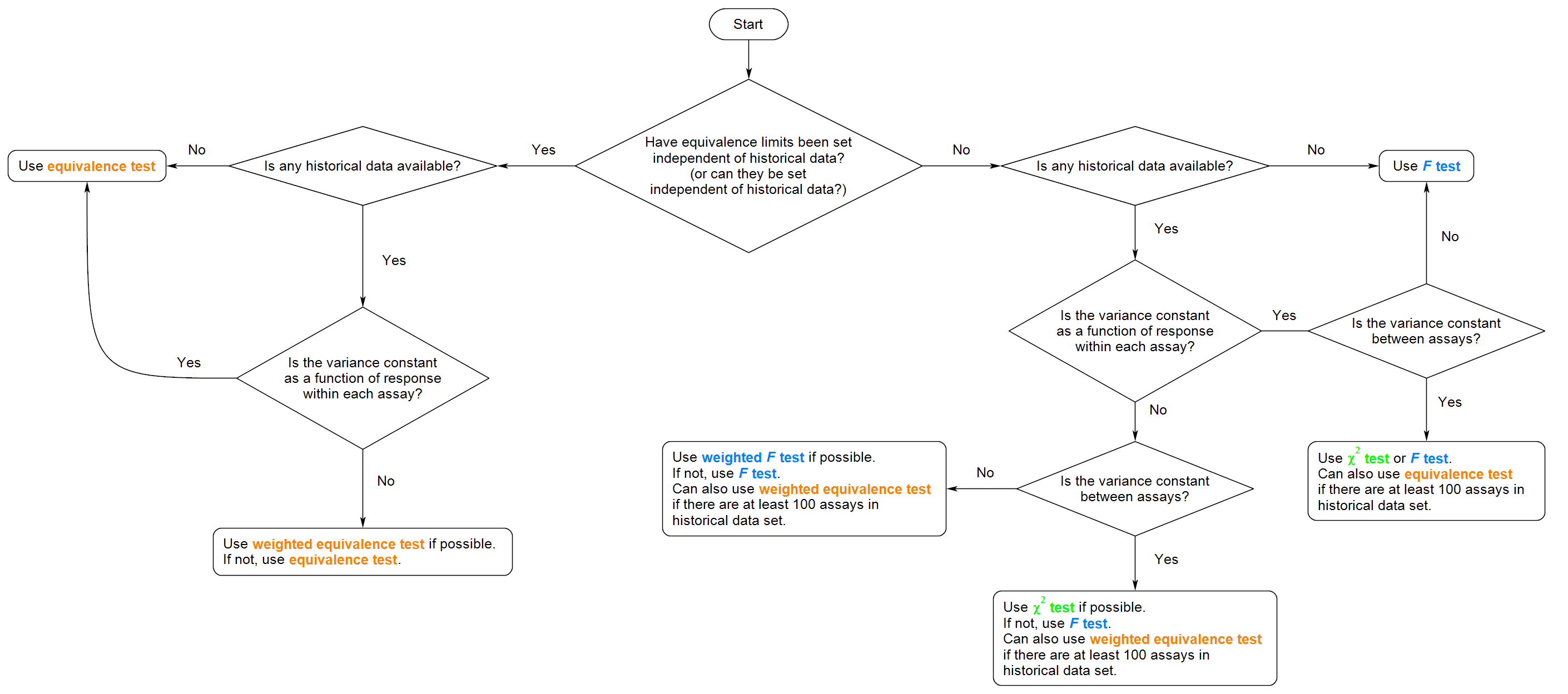Figure 10: Flow chart illustrating the best approach to testing parallelism in terms of reliability and practicality in a given situation.

When the equivalence limits for the equivalence test are based on ‘historical’ data we found this can easily result in the limits being set too high or too low. An alternative which avoids this problem is to set the limits independently of the data. This can lead to a larger false positive rate (as in scenario B). However, for fixed equivalence limits the false positive rate is the fraction of truly parallel assays with CIs for ∆ extending beyond the fixed equivalence limit. There is no reason to expect this fraction to be 5%, so this is not necessarily a problem.

# 8 Conclusions

Three common tests for parallelism in bioassays were reviewed: the F-test, the $$\chi^2$$-test and an equivalence test for the slope difference. The F-test is the simplest to apply and is applicable to all of the common models for bioassay data: linear models, non-linear models and generalized linear models. The $$\chi^2$$-test is more complicated because the variance of the data must be explicitly estimated to provide a weighting scheme for the model. For these two approaches the null hypothesis is that the standard and test products are similar. The equivalence test has the null hypothesis that the products are dissimilar. It is more complex to implement than the F-test because appropriate equivalence limits must be set.

It is important to note that there are several assumptions underlying all of these tests.  In the F-test the level of significance for the test is usually set at 5%, meaning that 5% of assays with true slope difference of zero will be rejected as dissimilar.  This takes no account of the importance of a given non-zero slope difference, and leads to the criticism of over-sensitivity of the F-test to small differences in precise assays. In practice the test could be adjusted to achieve a given probability of rejecting a given slope difference: this would be based on historical information about the within-assay variance.

The same is true of the $$\chi^2$$-test, and additionally an assumption is made that the variance is related to the mean response via a power law.

For the equivalence test, the equivalence interval must be set (often based on the probability that truly parallel assays will result in a confidence interval which is accepted) and the confidence level for the equivalence test must be chosen.

We examined the performance of the three tests via simulation of a linear model.  We found that both the $$\chi^2$$-test and the equivalence test outperformed the F-test on average, unless the assay-to-assay variation was considerable. If the amount of historical data available was limited to 20 assays, the performance of these approaches, especially the equivalence test based on historical data, was unreliable, varying from historical set to historical set. Historical data consisting of 100 assays provided a much more reliable performance for these two alternative approaches.

We have only considered a simple linear model in detail.  More complex models such as 4 or 5 parameter logistic models lead to more complexity, especially for the equivalence approach where limits must be set for 3 or 4 parameters respectively. We have investigated a simplified version of the 4 parameter logistic model, allowing only the slope parameter, but not the asymptotes, to be non-parallel; we found very similar results to the simple linear model.

There are no universal right or wrong ways to test for parallelism; only methods that may be more or less appropriate depending on the underlying beliefs about the similarity of the standard and test products, the precision of the data, the behaviour of the variance between and within assays, and the model applied to the data. The bioassay scientist and their statistician should decide on which approach to use by carefully considering the properties of the assay and the overall goals of the project, as indicated by the flow chart in Figure 10.

# References

Callahan JD, Sajjadi NC. 2003. Testing the null hypothesis for a specified difference – The right way to test for parallelism. Bioprocessing Journal, 2: 71-77.

Council of Europe. 2011. Statistical analysis of results of biological assays and tests. Pages 551-579 in European Pharmacopoeia, 7th ed. Council of Europe.

Finney DJ. 1964. Statistical Method in Biological Assay, 2nd ed.Griffin.

Gottschalk PG, Dunn JR. 2005a. Measuring parallelism, linearity, and relative potency in bioassay and immunoassay data. Journal of Biopharmaceutical Statistics, 15: 437-463.

Gottschalk PG, Dunn JR. 2005b. The five-parameter logistic: A characterization and comparison with the four-parameter logistic. Analytical biochemistry, 343: 54-65.

Hauck WW, Capen RC, Callahan JD, De Muth JE, Hsu H, Lansky D, Sajjadi NC, Seaver SS, Singer RR, Weisman D. Assessing parallelism prior to determining relative potency. PDA Journal of Pharmaceutical Science and Technology, 59: 127.

Jonkman JN, Sidik K. 2009. Equivalence Testing for Parallelism in the Four-Parameter Logistic Model. Journal of Biopharmaceutical Statistics, 19: 818-837.

McCullagh P, Nelder JA 1989.  Generalized Linear Models.  2nd Ed. Chapman and Hall, London

Novick SJ, Yang H, Peterson JJ. 2012. A Bayesian Approach to Parallelism Testing in Bioassay. Statistics in Biopharmaceutical Research, 4: 357-374

The United States Pharmacopeial Convention. 2012a. <1032> Design and development of biological assays. Pages 5160-5174 in First Supplement to USP 35-NF 30. The United States Pharmacopeial Convention.

The United States Pharmacopeial Convention. 2012b. <1033> Biological assay validation. Pages 5174-5185 in First Supplement to USP 35-NF 30. The United States Pharmacopeial Convention.

The United States Pharmacopeial Convention. 2012c. <1034> Analysis of biological assays. Pages 5186-5200 in First Supplement to USP 35-NF 30. The United States Pharmacopeial Convention.

Yang H, Zhang L. 2012. Evaluations of Parallelism Testing Methods Using ROC Analysis. Statistics in Biopharmaceutical Research, 4:162-173